# Rational Numbers As Quotients of Integers

##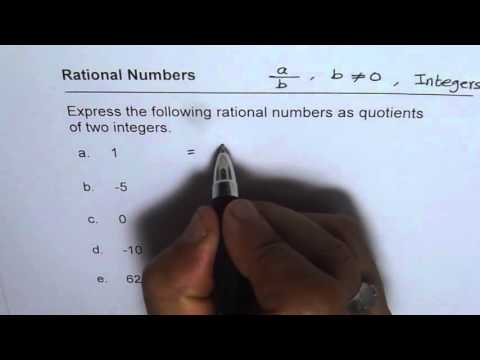By Anil Kumar

every quotient of integers is a rational number.# Division of Rational Numbers

##By Khan Academy

This video walks through the division of 4-5 rational numbers and includes a word problem.# Comparing rational numbers

##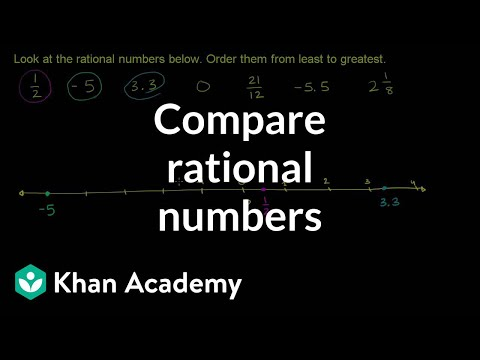By Khan Academy

U09_L1_T3_we4 Comparing Rational Numbers# Multiplying and dividing negative numbers

##By Khan Academy

Find the product of numbers with different signs.# Multiplying and dividing negative numbers

##By Khan Academy

Use the distributive property to understand the products of negative numbers.# Multiplying and dividing negative numbers

##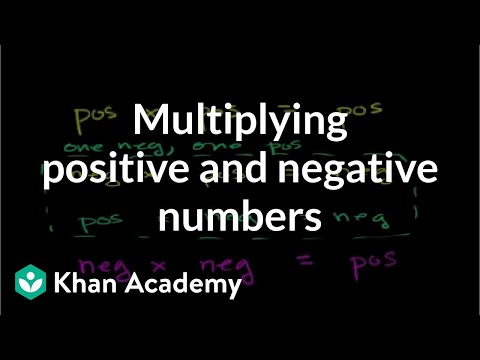By Khan Academy

Learn some rules of thumb for multiplying positive and negative numbers.# Multiplying and dividing negative numbers

##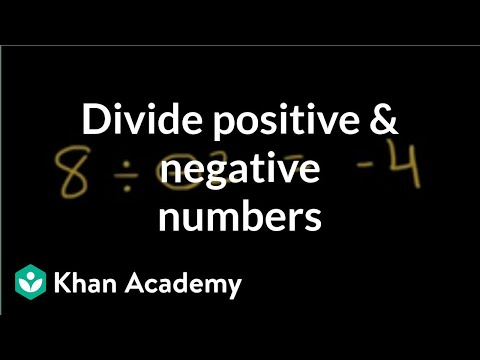By Khan Academy

Discover the basics of dividing with negative numbers.ï¿½ï¿½ï¿½ï¿½ï¿½ï¿½ï¿½ï¿½ï¿½ï¿½ï¿½ï¿½# Multiplying and dividing negative numbers

##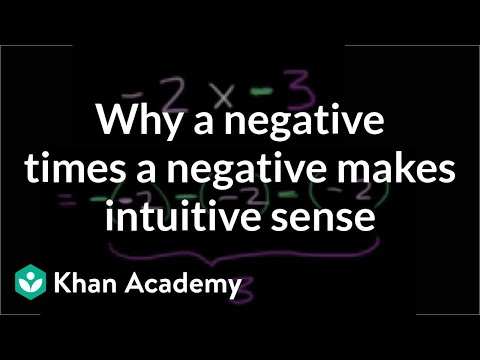By Khan Academy

Use the repeated addition model of multiplication to give an understanding of multiplying negative numbers.# Dividing mixed numbers

##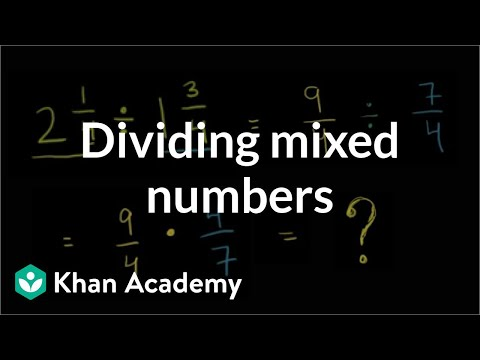By Khan Academy

Dividing Mixed Numbers# Order of operations with negative numbers

##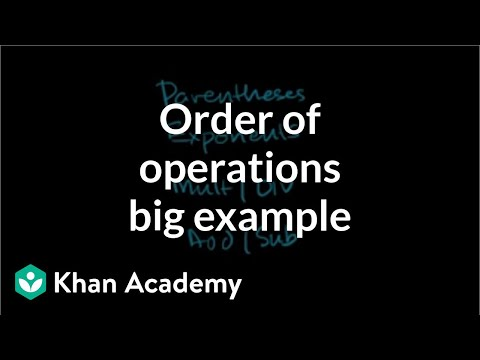By Khan Academy

Simplify this tricky expression using the order of operations. ï¿½ï¿½ï¿½ï¿½ï¿½ï¿½ï¿½ï¿½ï¿½ï¿½ï¿½ï¿½ Expression include negative numbers and exponents.# Order of operations with negative numbers

##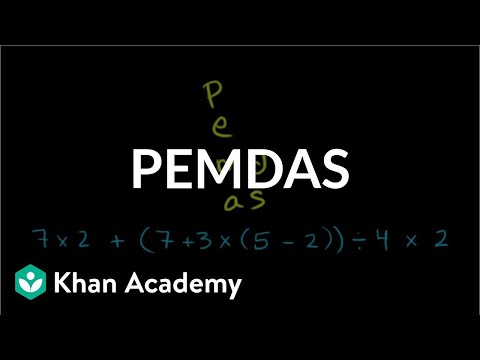By Khan Academy

Work through another challenging order of operations example with only positive numbers.# Order of operations with negative numbers

##By Khan Academy

This example clarifies the purpose of order of operations: to have ONE way to interpret a mathematical statement.# Fractional Exponent Expressions 2

##By Khan Academy

Fractional Exponent Expressions 2. All Khan Academy content is available for free at www.khanacademy.org# Comparing rational numbers

##By Khan Academy

Converting fractions to decimals sometimes requires us to brush up on our long division skills. We'll walk you through it.# Converting fractions to decimals

##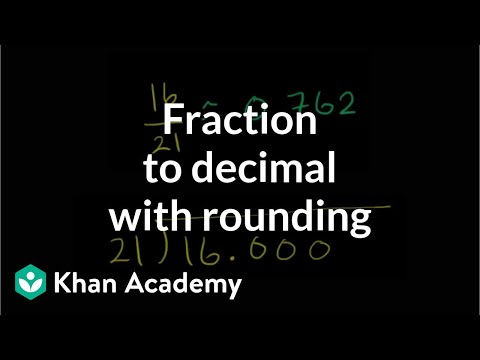By Khan Academy

Sometimes when you convert a fraction to a decimal you have to do some long division and rounding.# Converting fractions to decimals

##By Khan Academy

This is a great example of converting a fraction to a decimal. Use a scratch pad so you can follow along.# Converting fractions to decimals

##By Khan Academy

How to express a fraction as a decimal# 7.NS.A.1b / 7.NS.A.1c - Addition and Subtraction of Rational Numbers (Part 1 of 2)

##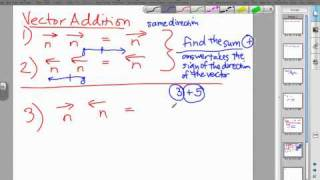By MathwithMrAlmeida

Watch Mr. Almeida fully explain how to add and subtract rational numbers using vectors and the concept of a number line.# Classifying numbers

##By Khan Academy

Learn how to classify numbers as whole numbers, integers, rational numbers, and irrational numbers.# Grade 7 Math - Multiplication & Division of Rational Numbers 5

##By Lumos Learning

Using the Lumos Study Programs, parents and educators can reinforce the classroom learning experience for children and help them succeed at school and on the standardized tests. Lumos books, dvd, eLearning and tutoring are used by leading schools, libraries and thousands of parents to supplement classroom learning and improve student achievement in the standardized tests.

PARENTS please visit LumosTestPrep.com to learn more.
EDUCATORS please visit LumosLearning.com to learn more